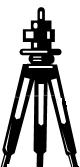* Log In to use the Calculate function * Become a Member!

###### Surveying & Earthwork Design:• Calculate distance by stadia measurement from survey rod readings and a level sighting.

• Calculate distance by stadia measurement from survey rod readings and an inclined sighting.
Instrument Height
• Calculate instrument height (HI) for a topo survey.
Point Elevations - Level Sight
• Calculate point elevations for a topo survey with a level sighting.
Point Elevations - Inclined Sight
• Calculate point elevations for a topo survey with an inclined sighting.
Volume - Average End Area
• Calculate earthwork volume by the average end area method.
Volume - Grid Square
• Calculate earthwork leveling by the grid square method (also called borrow pit leveling).
Traverse - Leg Distance
• Calculate the distance or length of the leg of a traverse.
Traverse - Leg Azimuth
• Calculate the azimuth of the leg of a traverse.
Traverse - Lat. and Dep.
• Calculate the length and departure to a vertice point of a traverse.
Traverse - Area by CM
• Calculate the area of a traverse by the coordinate method.
Earthwork Problem 1
• Calculate the storage volume of a proposed stormwater detention pond and the excavation haul volume given the soil bulking factor.

 Start Using The Calculations >>> Become A Member Now!